# Lecture II4 Filtering Sequential Estimation and the Ensemble

• Slides: 24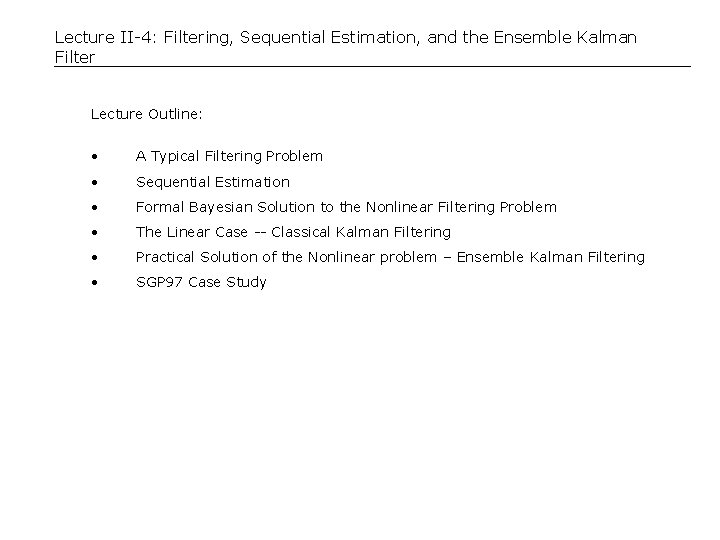Lecture II-4: Filtering, Sequential Estimation, and the Ensemble Kalman Filter Lecture Outline: • A Typical Filtering Problem • Sequential Estimation • Formal Bayesian Solution to the Nonlinear Filtering Problem • The Linear Case -- Classical Kalman Filtering • Practical Solution of the Nonlinear problem – Ensemble Kalman Filtering • SGP 97 Case Study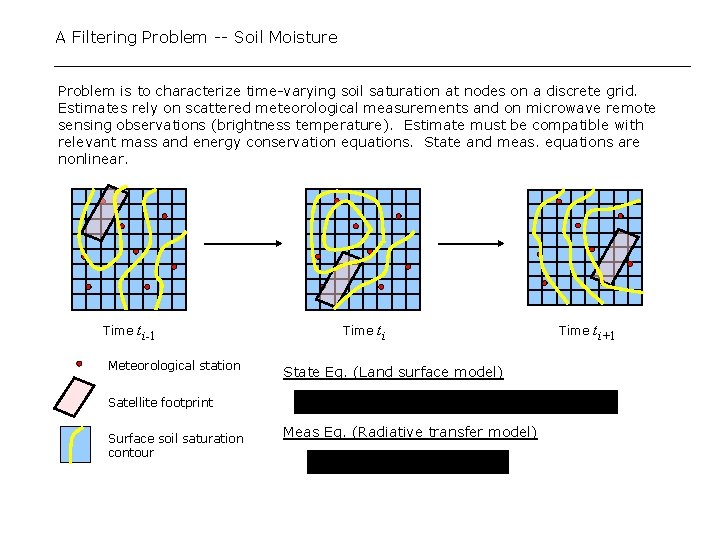A Filtering Problem -- Soil Moisture Problem is to characterize time-varying soil saturation at nodes on a discrete grid. Estimates rely on scattered meteorological measurements and on microwave remote sensing observations (brightness temperature). Estimate must be compatible with relevant mass and energy conservation equations. State and meas. equations are nonlinear. Time ti-1 Meteorological station Time ti State Eq. (Land surface model) Satellite footprint Surface soil saturation contour Meas Eq. (Radiative transfer model) Time ti+1Limitations of the Variational Approach for Filtering Applications Variational methods provide an effective way to estimate the mode of f y|z(y|z) for interpolation and smoothing problems. However, they have the following limitations: • The adjoint equations for a complex model may be difficult to derive, especially if the model includes discontinuous functions. • Most variational methods rely on gradient-based search algorithms which may have trouble converging. • Variational data assimilation algorithms tend to be complex, fragile, and difficult to implement, particularly for large time-dependent problems. • Variational algorithms generally provide no information on the likely range of system states around the conditional mode. • Most variational methods are unable to account for time-varying model input errors when problem size is large. Considering these limitations, it seems reasonable examine other alternatives for the filtering problem. One option is sequential estimation.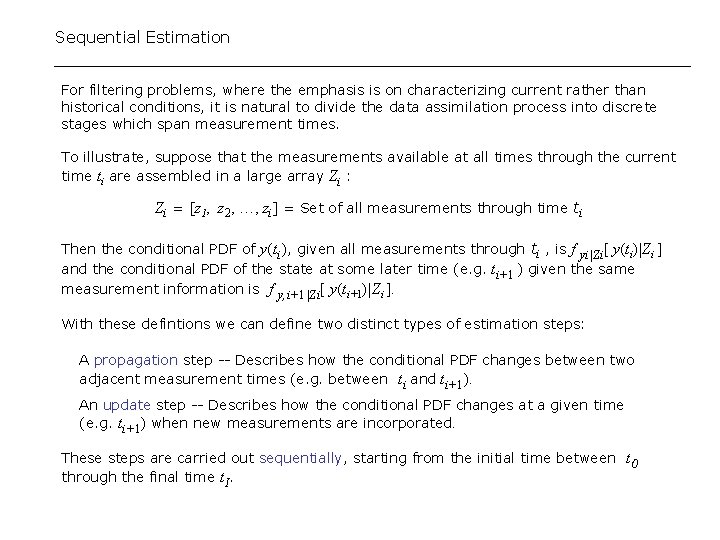Sequential Estimation For filtering problems, where the emphasis is on characterizing current rather than historical conditions, it is natural to divide the data assimilation process into discrete stages which span measurement times. To illustrate, suppose that the measurements available at all times through the current time ti are assembled in a large array Zi : Zi = [z 1, z 2, …, zi] = Set of all measurements through time ti Then the conditional PDF of y(ti), given all measurements through ti , is f yi|Zi[ y(ti)|Zi ] and the conditional PDF of the state at some later time (e. g. ti+1 ) given the same measurement information is f y, i+1|Zi[ y(ti+1)|Zi ]. With these defintions we can define two distinct types of estimation steps: A propagation step -- Describes how the conditional PDF changes between two adjacent measurement times (e. g. between ti and ti+1). An update step -- Describes how the conditional PDF changes at a given time (e. g. ti+1) when new measurements are incorporated. These steps are carried out sequentially, starting from the initial time between t 0 through the final time t. I.Propagating and Updating Conditional PDFs The propagation and update steps of the sequential estimation process are related as follows: Meas. 1 Meas. 2 z 1 z 2 Z 1 = [z 1 ] t 0 t 1 f y 1 [ y 1 ] zi Zi = [Zi-1 , zi ] Z 2 = [Z 1 , z 2 ] t 2 f y 2| z 1[ y 2|Z 1 ] i ti ti+1 f yi| zi-1[ yi|Zi-1 ] Propagation 1 to 2 Propagation 0 to 1 f y 0 [ y 0] Meas. f y 1| z 1[ y 1|Z 1 ] Update 1 Algorithm initialized with unconditional (prior) PDF at t 0 f y, i+1| zi[ yi+1|Zi ] Propagation i to i+1 f yi| zi[ yi|Zi ] Update i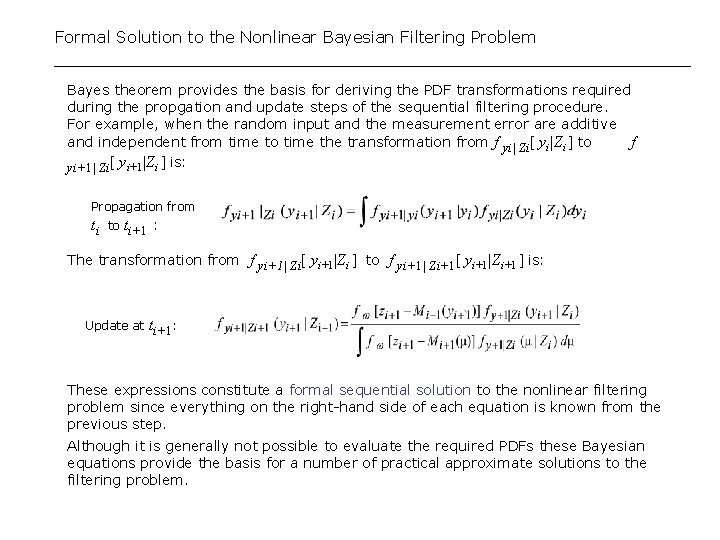Formal Solution to the Nonlinear Bayesian Filtering Problem Bayes theorem provides the basis for deriving the PDF transformations required during the propgation and update steps of the sequential filtering procedure. For example, when the random input and the measurement error are additive and independent from time to time the transformation from f yi| Zi[ yi|Zi ] to f yi+1| Zi[ yi+1|Zi ] is: Propagation from ti to ti+1 : The transformation from f yi+1| Zi[ yi+1|Zi ] to f yi+1| Zi+1[ yi+1|Zi+1 ] is: Update at ti+1: These expressions constitute a formal sequential solution to the nonlinear filtering problem since everything on the right-hand side of each equation is known from the previous step. Although it is generally not possible to evaluate the required PDFs these Bayesian equations provide the basis for a number of practical approximate solutions to the filtering problem.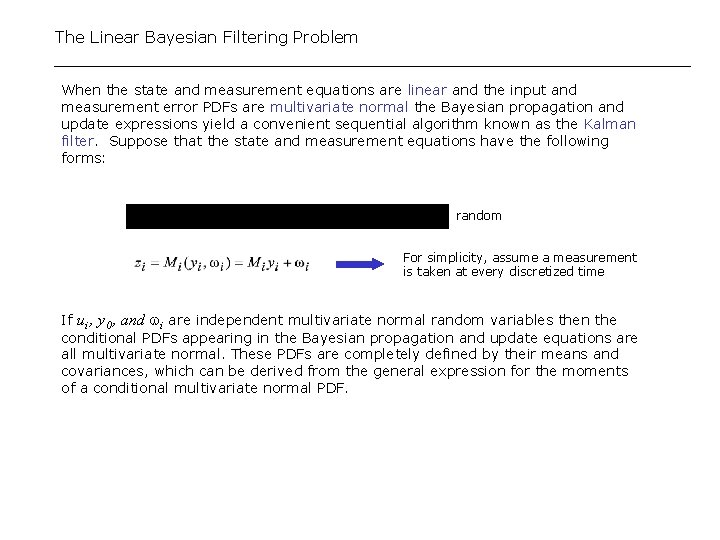The Linear Bayesian Filtering Problem When the state and measurement equations are linear and the input and measurement error PDFs are multivariate normal the Bayesian propagation and update expressions yield a convenient sequential algorithm known as the Kalman filter. Suppose that the state and measurement equations have the following forms: random For simplicity, assume a measurement is taken at every discretized time If ui , y 0 , and i are independent multivariate normal random variables then the conditional PDFs appearing in the Bayesian propagation and update equations are all multivariate normal. These PDFs are completely defined by their means and covariances, which can be derived from the general expression for the moments of a conditional multivariate normal PDF.The Classical Kalman Filter The conditional mean and covariance given by the Bayesian filter for the linear multivariate case are: Propagation from ti to ti+1 : Kalman gain Update at ti+1: forecast correction Note that the expression for the updated conditional mean (the estimate consists of two parts: • A forecast E(yi+1|Zi) based on data collected through ti • A correction term which depends on the difference between the forecast and the new measurement zi taken at ti The Kalman gain Ki+1 determines the weight given to the correction term. )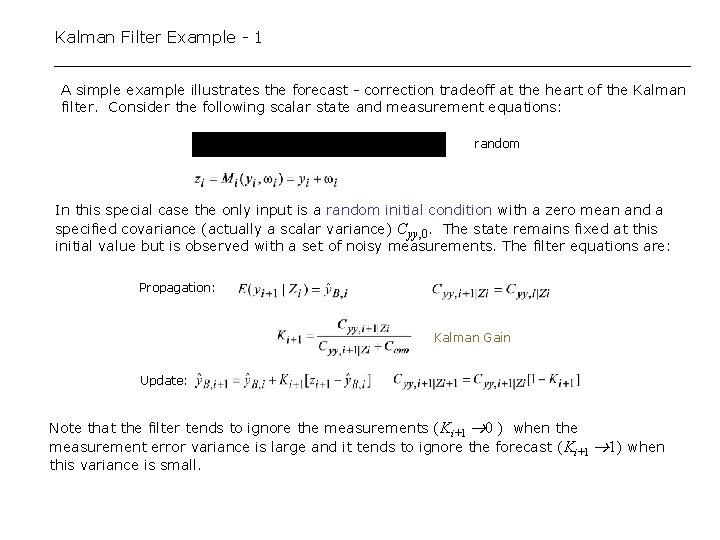Kalman Filter Example - 1 A simple example illustrates the forecast - correction tradeoff at the heart of the Kalman filter. Consider the following scalar state and measurement equations: random In this special case the only input is a random initial condition with a zero mean and a specified covariance (actually a scalar variance) Cyy, 0. The state remains fixed at this initial value but is observed with a set of noisy measurements. The filter equations are: Propagation: Kalman Gain Update: Note that the filter tends to ignore the measurements ( Ki+1 0 ) when the measurement error variance is large and it tends to ignore the forecast (Ki+1 1) when this variance is small.Updated estimate Kalman Filter Example - 2 2. 5 In this example the covariance and Kalman gain both decrease over time and the updated estimate converges to the true value of the unknown initial condition. The Kalman gain is smaller and the convergence is slower when the measurement error variance is larger. True value 2 1. 5 1 0. 5 0 20 40 60 80 100 0 Note that the Kalman gain approaches 1/t for large time. This implies that the estimate of the unknown initial condition is nearly equal to the sample mean of the measurements for the conditions specified here (variance of both initial condition and measurement error = 1. 0). 10 Kalman gain 1/t (Sample mean) -1 10 -2 10 0 20 40 60 Time 80 100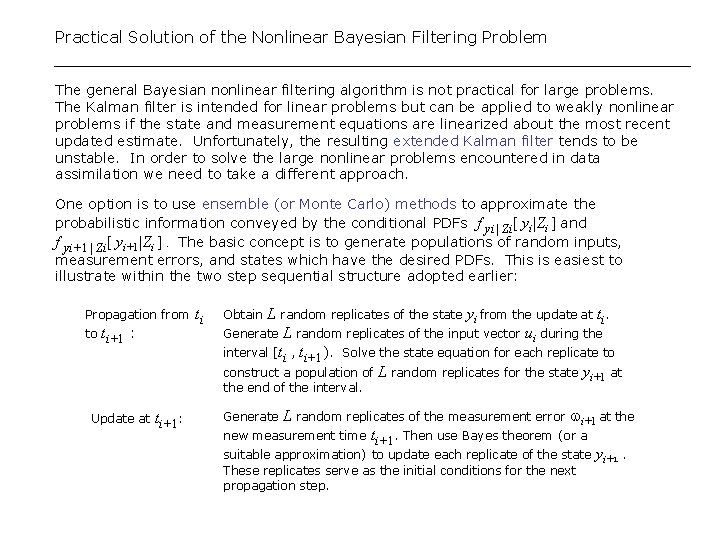Practical Solution of the Nonlinear Bayesian Filtering Problem The general Bayesian nonlinear filtering algorithm is not practical for large problems. The Kalman filter is intended for linear problems but can be applied to weakly nonlinear problems if the state and measurement equations are linearized about the most recent updated estimate. Unfortunately, the resulting extended Kalman filter tends to be unstable. In order to solve the large nonlinear problems encountered in data assimilation we need to take a different approach. One option is to use ensemble (or Monte Carlo) methods to approximate the probabilistic information conveyed by the conditional PDFs f yi| Zi[ yi|Zi ] and f yi+1| Zi[ yi+1|Zi ]. The basic concept is to generate populations of random inputs, measurement errors, and states which have the desired PDFs. This is easiest to illustrate within the two step sequential structure adopted earlier: Propagation from ti to ti+1 : L random replicates of the state yi from the update at ti. Generate L random replicates of the input vector ui during the interval [ti , ti+1). Solve the state equation for each replicate to construct a population of L random replicates for the state yi+1 at Obtain the end of the interval. Update at ti+1: L random replicates of the measurement error i+1 at the new measurement time ti+1. Then use Bayes theorem (or a suitable approximation) to update each replicate of the state yi+1. Generate These replicates serve as the initial conditions for the next propagation step.Ensemble filtering p(yi+1|Zi) Propagation of conditional probability density (formal Bayesian filtering): Propagate forward in time Update with new measurement (zi+1) p(yi+1|Zi+1) p(yi|Zi) ti ti+1 Evolution of random replicates in ensemble (Ensemble filtering): Time Update with new measurement y l(ti+1| Zi+1) y l(ti| Zi) Propagate forward in time ti y l(ti+1| Zi) ti+1 Time Ensemble filtering propagates only replicates (no PDFs). But how should update be performed? It is not practical to construct complete multivariate PDF and update with Bayes theorem.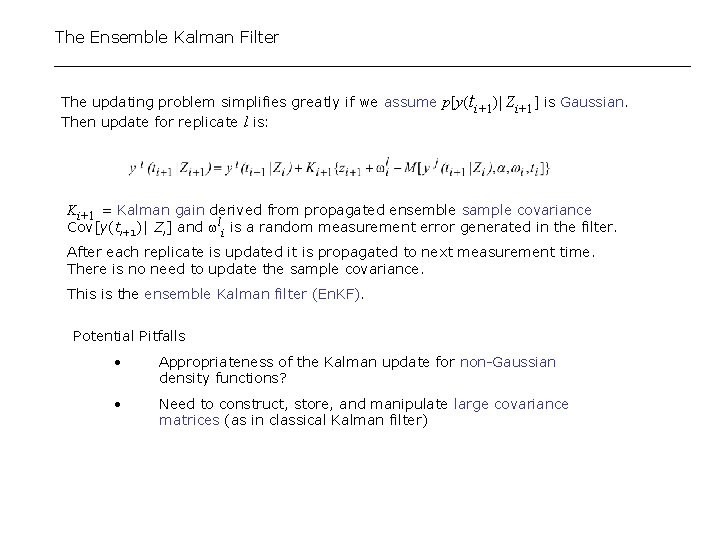The Ensemble Kalman Filter The updating problem simplifies greatly if we assume p[y(ti+1)| Zi+1] is Gaussian. Then update for replicate l is: Ki+1 = Kalman gain derived from propagated ensemble sample covariance Cov[y(ti+1)| Zi] and li is a random measurement error generated in the filter. After each replicate is updated it is propagated to next measurement time. There is no need to update the sample covariance. This is the ensemble Kalman filter (En. KF). Potential Pitfalls • Appropriateness of the Kalman update for non-Gaussian density functions? • Need to construct, store, and manipulate large covariance matrices (as in classical Kalman filter)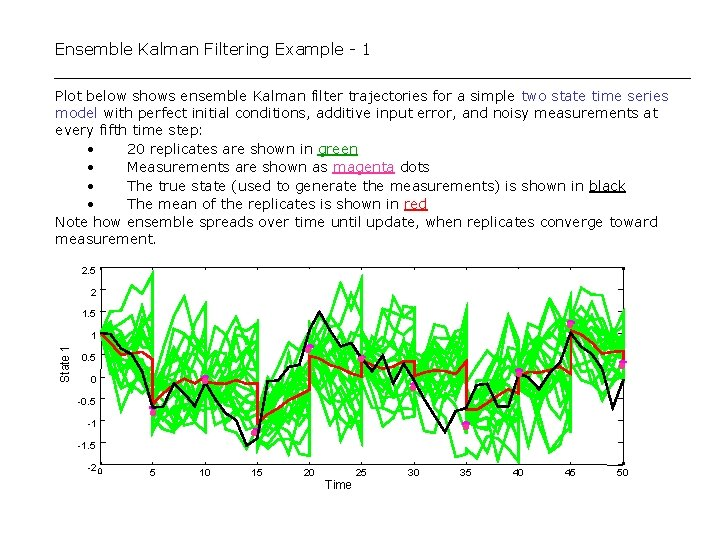Ensemble Kalman Filtering Example - 1 Plot below shows ensemble Kalman filter trajectories for a simple two state time series model with perfect initial conditions, additive input error, and noisy measurements at every fifth time step: • 20 replicates are shown in green • Measurements are shown as magenta dots • The true state (used to generate the measurements) is shown in black • The mean of the replicates is shown in red Note how ensemble spreads over time until update, when replicates converge toward measurement. 2. 5 2 1. 5 State 1 1 • 0. 5 -1 • 5 10 15 • • • -1. 5 -2 0 • • 0 -0. 5 • 20 25 Time 30 35 40 45 50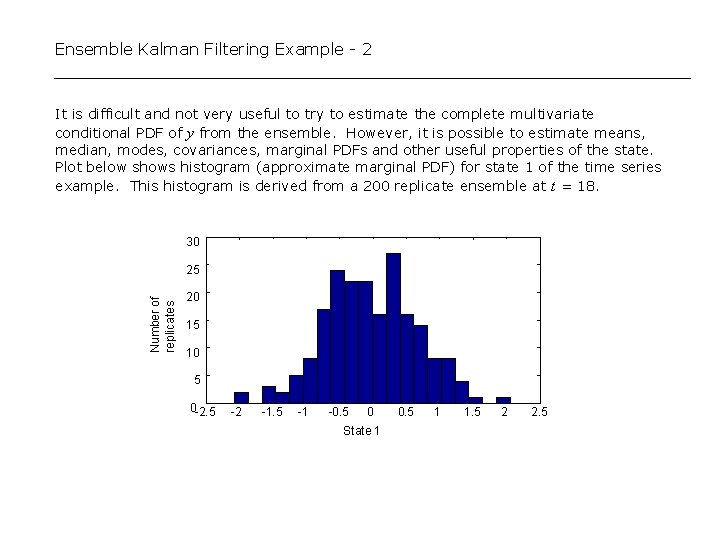Ensemble Kalman Filtering Example - 2 It is difficult and not very useful to try to estimate the complete multivariate conditional PDF of y from the ensemble. However, it is possible to estimate means, median, modes, covariances, marginal PDFs and other useful properties of the state. Plot below shows histogram (approximate marginal PDF) for state 1 of the time series example. This histogram is derived from a 200 replicate ensemble at t = 18. 30 Number of replicates 25 20 15 10 5 0 -2. 5 -2 -1. 5 -1 -0. 5 0 State 1 0. 5 1 1. 5 2 2. 5SGP 97 Experiment - Soil Moisture Campaign Case Study Area Aircraft microwave measurements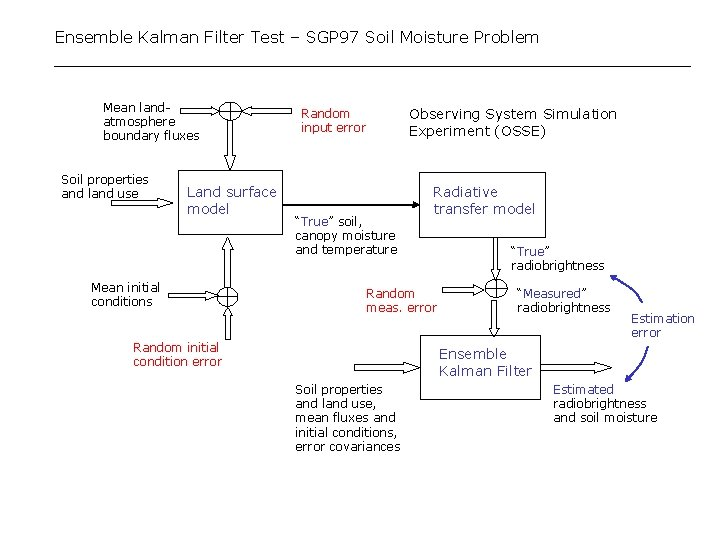Ensemble Kalman Filter Test – SGP 97 Soil Moisture Problem Mean landatmosphere boundary fluxes Soil properties and land use Land surface model Mean initial conditions Observing System Simulation Experiment (OSSE) Random input error “True” soil, canopy moisture and temperature Radiative transfer model Random meas. error Random initial condition error “True” radiobrightness “Measured” radiobrightness Estimation error Ensemble Kalman Filter Soil properties and land use, mean fluxes and initial conditions, error covariances Estimated radiobrightness and soil moisture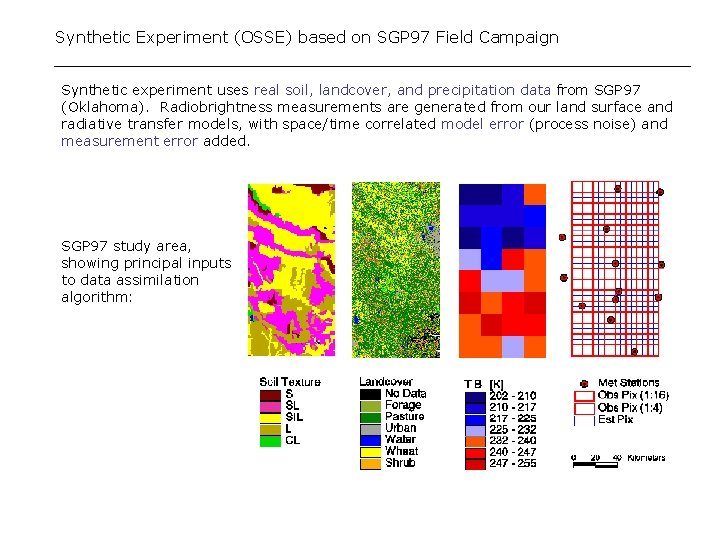Synthetic Experiment (OSSE) based on SGP 97 Field Campaign Synthetic experiment uses real soil, landcover, and precipitation data from SGP 97 (Oklahoma). Radiobrightness measurements are generated from our land surface and radiative transfer models, with space/time correlated model error (process noise) and measurement error added. SGP 97 study area, showing principal inputs to data assimilation algorithm: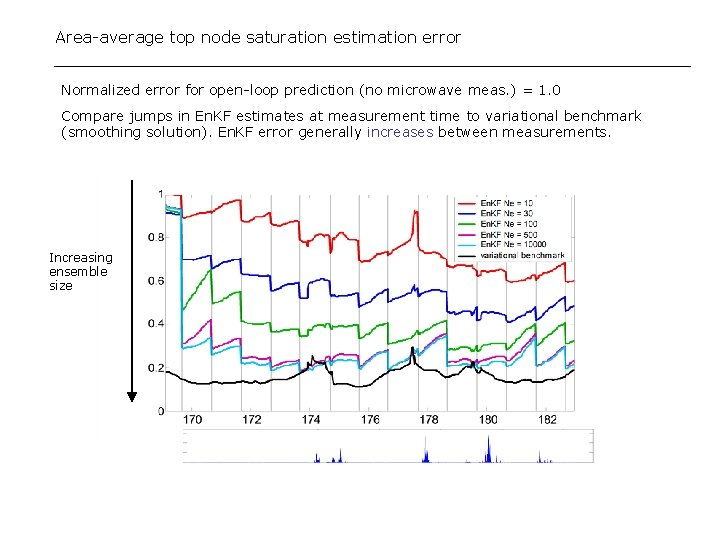Area-average top node saturation estimation error Normalized error for open-loop prediction (no microwave meas. ) = 1. 0 Compare jumps in En. KF estimates at measurement time to variational benchmark (smoothing solution). En. KF error generally increases between measurements. Increasing ensemble size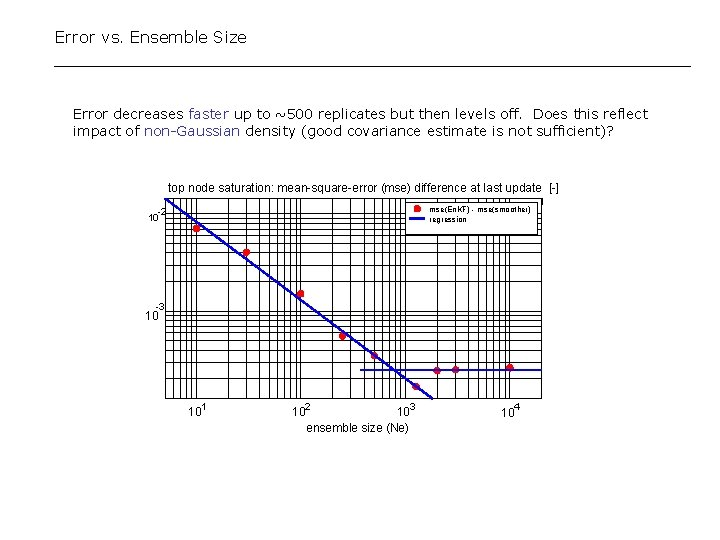Error vs. Ensemble Size Error decreases faster up to ~500 replicates but then levels off. Does this reflect impact of non-Gaussian density (good covariance estimate is not sufficient)? top node saturation: mean-square-error (mse) difference at last update [-] mse(En. KF) - mse(smoother) regression -2 10 -3 10 1 10 2 10 103 ensemble size (Ne) 4 10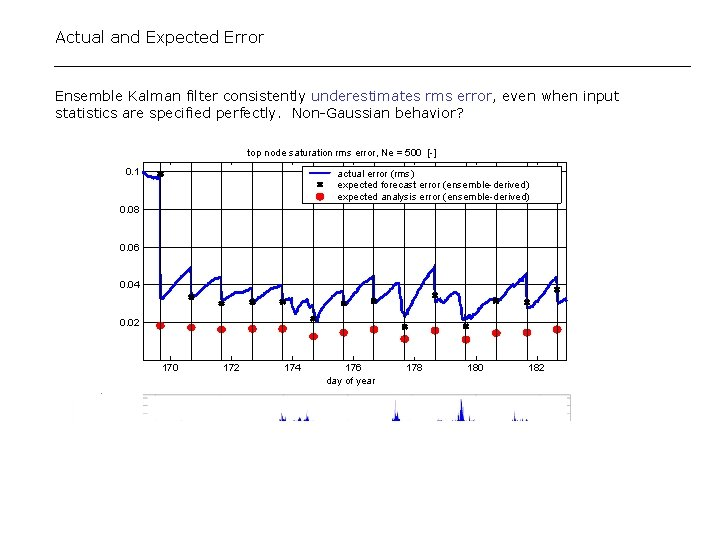Actual and Expected Error Ensemble Kalman filter consistently underestimates rms error, even when input statistics are specified perfectly. Non-Gaussian behavior? top node saturation rms error, Ne = 500 [-] 0. 1 actual error (rms) expected forecast error (ensemble-derived) expected analysis error (ensemble-derived) 0. 08 0. 06 0. 04 0. 02 170 172 174 176 day of year 178 180 182Ensemble Error Distribution Errors appear to be more Gaussian at intermediate moisture values and more skewed at high or low values. Uncertainty is small just after a storm, grows with drydown, and decreases again when soil is very dry. pixel 283: ensemble of top node saturation 0 5 10 0. 7 forecast Ne = 500 analysis Ne = 500 15 0. 5 precipitation [mm/h] top node saturation [-] 0. 6 0. 4 0. 3 0. 2 0. 1 0 1 2 3 4 5 6 7 8 9 observation time 10 11 12 13 14Model Error Estimates Ensemble Kalman filter provides discontinuous but generally reasonable estimates of model error but sample problem. Compare to smoothed error estimate from variational benchmark. Specified error statistics are perfect. pixel 283: model error in moisture flux upper b. c. [mm/d] 5 open loop true En. KF Ne = 500 variational benchmark 4 3 2 1 0 -1 -2 170 172 174 176 day of year 178 180 182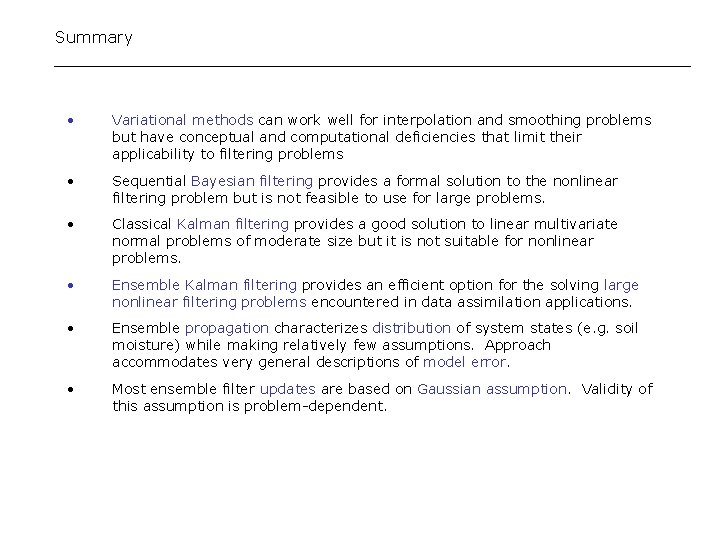Summary • Variational methods can work well for interpolation and smoothing problems but have conceptual and computational deficiencies that limit their applicability to filtering problems • Sequential Bayesian filtering provides a formal solution to the nonlinear filtering problem but is not feasible to use for large problems. • Classical Kalman filtering provides a good solution to linear multivariate normal problems of moderate size but it is not suitable for nonlinear problems. • Ensemble Kalman filtering provides an efficient option for the solving large nonlinear filtering problems encountered in data assimilation applications. • Ensemble propagation characterizes distribution of system states (e. g. soil moisture) while making relatively few assumptions. Approach accommodates very general descriptions of model error. • Most ensemble filter updates are based on Gaussian assumption. Validity of this assumption is problem-dependent.